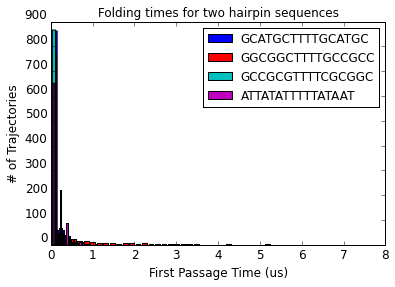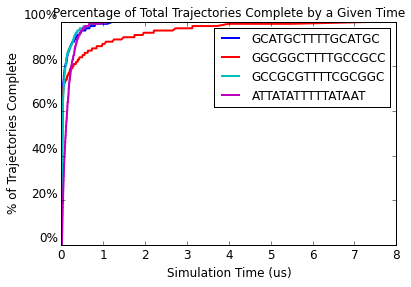# Tutorial 4 - Hairpin first passage time¶

Here we ask Multistrand to simulate the folding of an open strand into a hairpin, and stop when it's done. This mode, First Passage Time Mode, requires us to specific a start state and a recognizable stop condition (i.e. entering a macrostate), and Multistrand returns the total time it took. Running a lot of simulations and plotting histograms and completion fractions can provide insight into the process -- and its stochastic variability.

In :
import numpy as np
import matplotlib
import matplotlib.pylab as plt
%matplotlib inline

from multistrand.objects import *
from multistrand.options import Options
from multistrand.system import SimSystem


#### This is first passage time¶

In :
# for StopCondition and Macrostate definitions:
Exact_Macrostate = 0   # match a secondary structure exactly (i.e. any system state that has a complex with this exact structure)
Bound_Macrostate = 1   # match any system state in which the given strand is bound to another strand
Dissoc_Macrostate = 2  # match any system state in which there exists a complex with exactly the given strands, in that order
Loose_Macrostate = 3   # match a secondary structure with "don't care"s, allowing a certain number of disagreements
Count_Macrostate = 4   # match a secondary structure, allowing a certain number of disagreements

In :
def setup_options_hairpin(trials, stem_seq, hairpin_seq):

# Define the domains
stem = Domain(name="stem",sequence=stem_seq,length=len(stem_seq))
hairpin = Domain(name="hairpin", sequence=hairpin_seq,length=len(hairpin_seq))
s = stem + hairpin + stem.C

# We give domain-level structures for the open and closed hairpin configurations
start_complex = Complex(strands=[s], structure="...")
stop_complex  = Complex(strands=[s], structure="(.)")
full_sc       = StopCondition( "CLOSED", [(stop_complex,Exact_Macrostate,0)])
# Note: unlike in Transition Mode, in First Passage Time Mode, no "stop:" prefix is needed in the macrostate name
# in order for the StopCondition to trigger the end of the simulation.

o = Options(simulation_mode="First Passage Time",parameter_type="Nupack",substrate_type="DNA", temperature=310.15,
num_simulations = trials, simulation_time=0.1, rate_scaling='Calibrated', verbosity=0,
start_state=[start_complex], stop_conditions=[full_sc])

return o


The simulation is easy. There's more fuss and bother to define how to plot the results.

In :
def plot_histograms( result_lists, colors=['b','r','c','m','g','k'], figure=1, labels=None ):
times = []
for n in range(len(result_lists)):
times.append(1e6* np.array([ i.time for i in result_lists[n] ]))    # convert from seconds to microseconds units.

min_time = np.min( [np.min(times[n]) for n in range(len(result_lists)) ] )
max_time = np.max( [np.max(times[n]) for n in range(len(result_lists)) ] )

plt.figure( figure )
plt.hold(False)
for n in range(len(result_lists)):
plt.hist( times[n], 50, range=(min_time,max_time), color = colors[n], label=labels[n], rwidth=(1-n*1.0/len(result_lists)) )
plt.hold(True)

plt.title("Folding times for two hairpin sequences")
plt.xlabel("First Passage Time (us)",fontsize='larger')
plt.ylabel("# of Trajectories",fontsize='larger')
plt.yticks(fontsize='larger',va='bottom')
plt.xticks(fontsize='larger')
plt.legend(loc=0)
plt.show()

def plot_completion_graph( result_lists, colors=['b','r','c','m','g','k'], figure=1, labels=None ):
times = []
percents = []
if labels==None:
labels=[str(n) for n in range(1,1+len(result_lists))]
if len(labels) < len(colors):
colors = colors[:len(labels)]

for rl in result_lists:
n = len(rl)
t = [i.time for i in rl]
t.sort()
times.append(np.array(t))

p = np.array(range(1,len(t)+1))
p = 100 * p / n  # percentage of all trials
percents.append( p )

plt.figure( figure )
plt.hold(False)

for t,p,c,label in zip(times,percents,colors,labels):
plt.plot( 1e6 * t, p, color = c, linewidth=2.0, label=label )
plt.hold(True)

plt.xlabel("Simulation Time (us)",fontsize='larger')
plt.ylabel("% of Trajectories Complete",fontsize='larger')
plt.yticks([0,20,40,60,80,100],("0%","20%","40%","60%","80%","100%"),fontsize='larger',va='bottom')
plt.xticks(fontsize='larger')
plt.title( "Percentage of Total Trajectories Complete by a Given Time" )
plt.legend(loc=0)
plt.show()

In :
# First Passage Time Mode doesn't store the full trajectory.  But it gives the random number seed used on a given simulation.
# So we can re-run interesting simulations to see what happened.
# Here, we also show how to run a Trajectory Mode simulation with a StopCondition (rather than time-out).
def show_interesting_trajectories( result_lists, seqs, type='fastest' ):
mintimes = []
maxtimes = []
slowseeds = []
fastseeds = []
for n in range(len(result_lists)):
times = 1e6* np.array([ i.time for i in result_lists[n] ])    # convert from seconds to microseconds units.
slowseeds.append( result_lists[n][np.argmax( times )].seed )  # find the seed used for the slowest simulation
fastseeds.append( result_lists[n][np.argmin( times )].seed )  # find the seed used for the fastest simulation
mintimes.append( np.min( times ) )
maxtimes.append( np.max( times ) )

# taken from hairpin_trajectories.py
def print_trajectory(o):
print o.full_trajectory   # the strand sequence
print o.start_state.structure
for i in range(len(o.full_trajectory)):
time = 1e6* o.full_trajectory_times[i]
state = o.full_trajectory[i]
struct = state
dG = state
print struct + ' t=%11.9f microseconds, dG=%6.2f kcal/mol' % (time, dG)

# take a look at the fastest folds.  to take a look at the more interesting slowest folds,
# change "mintimes" to "maxtimes" and change "fastseeds" to "slowseeds"; do this based on 'type' argument
seeds = fastseeds if type=='fastest' else slowseeds
times = mintimes if type=='fastest' else maxtimes
for (seq,seed,time) in zip(seqs,seeds,times):
s1 = Strand(name="hairpin", sequence=seq)
c1 = Complex( strands=[s1], structure=16*'.' )             # hard-coded length 16
c2 = Complex( strands=[s1], structure="((((((....))))))")  # hard-coded stem length 6, loop length 4
sc  = StopCondition( "CLOSED", [(c2,Exact_Macrostate,0)])
# For future reference, StopConditions and Macrostates (same thing) are provided as a list of match conditions,
# all of which must be matched.  I.e. there is an implicit AND being evaluated.  E.g.
# sc = StopCondition( "EXAMPLE", [(c2,Loose_Macrostate,8), (c1,Loose_Macrostate,4)]
# would specify the intersection of those two macrostates, i.e. any 2 base pairs of the helix (and maybe more).

o = Options(temperature=310.15, dangles='Some', start_state = [c1],
simulation_time = 0.1,  # 0.1 seconds  (lots more time than hairpin_trajectories, to accommodate slow folds)
num_simulations = 1,  # don't play it again, Sam
output_interval = 1,  # record every single step
rate_method = 'Metropolis', # the default is 'Kawasaki' (numerically, these are 1 and 2 respectively)
rate_scaling = 'Calibrated', # this is the same as 'Default'.  'Unitary' gives values 1.0 to both.
o.stop_conditions=[sc]               # don't wait for the time-out
o.initial_seed = seed                # start with the same random seed as before...

s = SimSystem(o)
s.start()
print_trajectory(o)
print "Original run's time: %g microseconds" % time

In :
def run_sims():
print "Performing simulations..."
# compare closing of stems based on possible kinetic traps
o1 = setup_options_hairpin(trials=1000, stem_seq = "GCATGC", hairpin_seq="TTTT")  # 2-base misaligned GC pairing possible
o2 = setup_options_hairpin(trials=1000, stem_seq = "GGCGGC", hairpin_seq="TTTT")  # 3-base misaligned GGC pairing possible
o3 = setup_options_hairpin(trials=1000, stem_seq = "GCCGCG", hairpin_seq="TTTT")  # strong stem, but no significant misaligned pairing
o4 = setup_options_hairpin(trials=1000, stem_seq = "ATTATA", hairpin_seq="TTTT")  # weak stem, no significant misaligned pairing

s=SimSystem(o1)
s.start()
s=SimSystem(o2)
s.start()
s=SimSystem(o3)
s.start()
s=SimSystem(o4)
s.start()
# all these simulations are at the same join_concentration and temperature,
# so there's no need to re-initialize the energy model before each one.

all_results = [o.interface.results for o in [o1, o2, o3, o4]]
all_seqs    = [o.start_state.sequence for o in [o1, o2, o3, o4]]

print "Plotting results..."
plot_histograms(all_results, figure=1, labels=all_seqs)
plot_completion_graph(all_results, figure=2, labels=all_seqs)
# conclusions:
#    weak stem forms slowly, but without traps.
#    stronger stems form quickly, but if 3-base misalignment is possible, it may take a long time

special = 'fastest'   # or 'slowest', if you wish, but these trajectories involve many many steps!
print "Showing the %s trajectories for each hairpin sequence..." % special
show_interesting_trajectories(all_results, all_seqs, special)


OK, time to actually run the simulations.

In :
run_sims()

Performing simulations...
Plotting results...Showing the fastest trajectories for each hairpin sequence...
GCATGCTTTTGCATGC
................
(..............) t=0.001031155 microseconds, dG=  3.60 kcal/mol
((............)) t=0.001045078 microseconds, dG=  1.26 kcal/mol
(((..........))) t=0.001374474 microseconds, dG= -0.17 kcal/mol
(((.(......).))) t=0.001512538 microseconds, dG=  0.31 kcal/mol
(((.((....)).))) t=0.001559573 microseconds, dG= -2.83 kcal/mol
((((((....)))))) t=0.001590837 microseconds, dG= -6.16 kcal/mol
Original run's time: 0.00159084 microseconds
GGCGGCTTTTGCCGCC
................
.....(....)..... t=0.000096955 microseconds, dG=  1.15 kcal/mol
..(..(....)..).. t=0.000260229 microseconds, dG=  1.85 kcal/mol
.((..(....)..)). t=0.000565808 microseconds, dG= -0.31 kcal/mol
.((.((....)).)). t=0.000601655 microseconds, dG= -2.25 kcal/mol
.(((((....))))). t=0.000699386 microseconds, dG= -7.26 kcal/mol
((((((....)))))) t=0.000975618 microseconds, dG= -8.23 kcal/mol
Original run's time: 0.000975618 microseconds
GCCGCGTTTTCGCGGC
................
..(..........).. t=0.000101750 microseconds, dG=  2.34 kcal/mol
.((..........)). t=0.000572517 microseconds, dG=  0.51 kcal/mol
.(((........))). t=0.000575432 microseconds, dG= -1.66 kcal/mol
.((((......)))). t=0.000790635 microseconds, dG= -4.20 kcal/mol
(((((......))))) t=0.001174522 microseconds, dG= -5.49 kcal/mol
((((((....)))))) t=0.001186023 microseconds, dG= -8.56 kcal/mol
Original run's time: 0.00118602 microseconds
Count: 10 Time: 3.22893301395e-09
ATTATATTTTTATAAT
................
.(...........).. t=0.000810654 microseconds, dG=  3.53 kcal/mol
.(..(......).).. t=0.001034812 microseconds, dG=  5.33 kcal/mol
.(..((....)).).. t=0.001108170 microseconds, dG=  3.95 kcal/mol
....((....)).... t=0.001267316 microseconds, dG=  1.70 kcal/mol
(...((....)))... t=0.001572158 microseconds, dG=  4.79 kcal/mol
....((....)).... t=0.001696162 microseconds, dG=  1.70 kcal/mol
..(.((....)).).. t=0.001803105 microseconds, dG=  2.85 kcal/mol
.((.((....)).)). t=0.002172892 microseconds, dG=  1.70 kcal/mol
.(((((....))))). t=0.002200039 microseconds, dG= -0.76 kcal/mol
((((((....)))))) t=0.003228933 microseconds, dG= -1.27 kcal/mol
Original run's time: 0.00322893 microseconds

In [ ]: